5. vector-raster conversions, reprojection, warping

For a better version of the stars vignettes see https://r-spatial.github.io/stars/articles/

This vignette shows how stars object can be moved from vector and raster representations.

Rasterizing an sf vector object

library(stars)
system.file("gpkg/nc.gpkg", package = "sf") %>%
st_transform(32119) -> nc
nc$dens = nc$BIR79 / units::set_units(st_area(nc), km^2)
(nc.st = st_rasterize(nc["dens"], dx = 5000, dy = 5000))
## stars object with 2 dimensions and 1 attribute
## attribute(s):
##                    Min.  1st Qu.   Median     Mean  3rd Qu.     Max. NA's
## dens [1/km^2] 0.2545072 1.225631 1.932222 3.345918 3.825745 21.24828 4808
## dimension(s):
##   from  to offset delta                 refsys point values x/y
## x    1 162 123829  5000 NAD83 / North Carolina FALSE   NULL [x]
## y    1  61 318260 -5000 NAD83 / North Carolina FALSE   NULL [y]
plot(nc.st)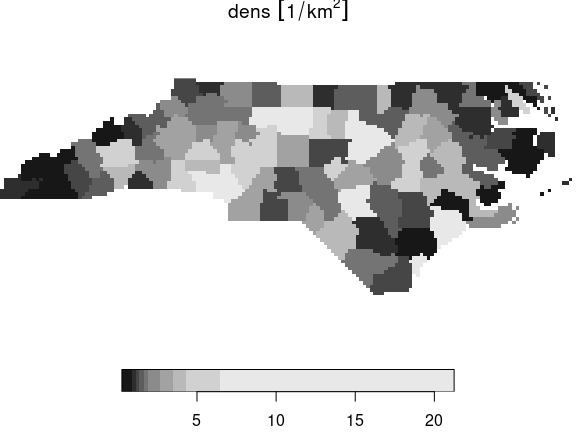The algorithm used is the GDAL rasterize utility, all options of this utility can be passed to st_rasterize. The geometry of the final raster can be controlled by passing a target bounding box and either the raster dimensions nx and ny, or pixel size by the dx and dy parameters.

Vectorizing a raster object to an sf object

stars objects can be converted into an sf object using st_as_sf. It has a number of options, depending on whether pixels represent the point value at the pixel center, or small square polygons with a single value.

We will work again with the landsat-7 6-band image, but will select the first band and round the values:

tif = system.file("tif/L7_ETMs.tif", package = "stars")
x = read_stars(tif)[, 1:50, 1:50, 1:2]
x[] = round(x[]/5)

Polygonizing

In case raster cells reflect point values and we want to get a vector representation of the whole field, we can draw contour lines and export the contour sets (only available when the GDAL version is at least 2.4.0):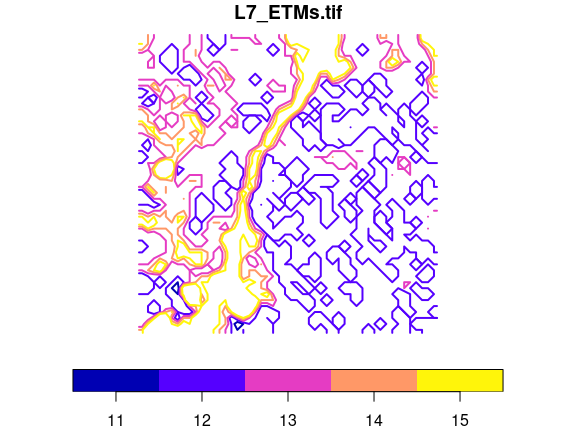Exporting to polygons

Alternatively, we can export to polygons and either get a single polygon per pixel, as in

or merge polygons that have identical pixel values;

When plotted with boundaries, we see the resolved boundaries of areas with the same pixel value: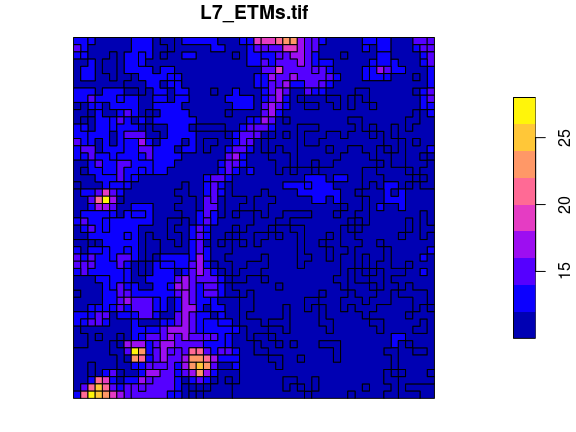A further option connect8 can be set to TRUE to use 8 connectedness, rather than the default 4 connectedness algorithm. In both cases, the polygons returned will often be invalid according to the simple feature standard, but can be made valid using lwgeom::st_make_valid.

Switching between vector and raster in stars objects

We can convert a raster dimension to a vector dimension while keeping other dimensions as they are in a stars object by

x.sf = st_xy2sfc(x, as_points = TRUE)
x.sf
## stars object with 2 dimensions and 1 attribute
## attribute(s):
##              Min. 1st Qu. Median    Mean 3rd Qu. Max.
## L7_ETMs.tif     7       9     11 11.2548      12   28
## dimension(s):
##          from   to offset delta                     refsys point
## geometry    1 2500     NA    NA SIRGAS 2000 / UTM zone 25S  TRUE
## band        1    2     NA    NA                         NA    NA
##                                                       values
## geometry POINT (288790.5 9120747),...,POINT (290187 9119350)
## band                                                    NULL

which also requires setting the as_points arguments as in st_as_sf.

Reprojecting a raster

If we accept that curvilinear rasters are rasters too, and that regular and rectilinear grids are special cases of curvilinear grids, reprojecting a raster is no longer a “problem”, it just recomputes new coordinates for every raster cell, and generally results in a curvilinear grid (that sometimes can be brought back to a regular or rectilinear grid). If curvilinear grid cells are represented by coordinates of the cell center, the actual shape of a grid cell gets lost, and this may be a larger effect if grid cells are large or if the transformation is stronger non-linear.

An example of the reprojection of the grid created above is

nc.st %>% st_transform("+proj=laea +lat_0=34 +lon_0=-60") -> nc.curv
nc.curv
## stars object with 2 dimensions and 1 attribute
## attribute(s):
##                    Min.  1st Qu.   Median     Mean  3rd Qu.     Max. NA's
## dens [1/km^2] 0.2545072 1.225631 1.932222 3.345918 3.825745 21.24828 4808
## dimension(s):
##   from  to offset delta                       refsys point
## x    1 162     NA    NA +proj=laea +lat_0=34 +lon... FALSE
## y    1  61     NA    NA +proj=laea +lat_0=34 +lon... FALSE
##                           values x/y
## x [162x61] -2210936,...,-1371611 [x]
## y    [162x61] 90650.2,...,538204 [y]
## curvilinear grid
plot(nc.curv, border = NA, graticule = TRUE)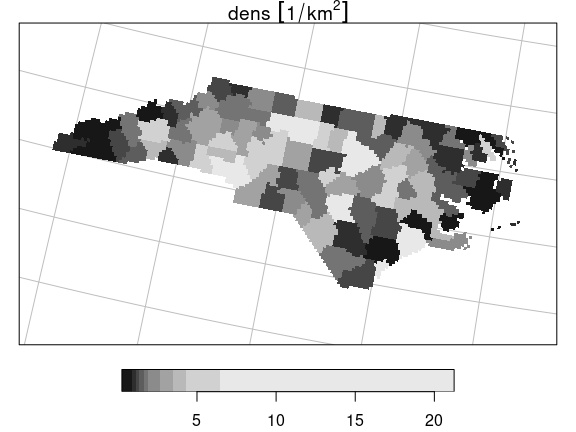where it should be noted that the dimensionality of the grid didn’t change: the same set of raster cells has been replotted in the new CRS, but now in a curvilinear grid.

Warping a raster

Warping a raster means creating a new regular grid in a new CRS, based on a (usually regular) grid in another CRS. We can do the transformation of the previous section by first creating a target grid:

nc %>% st_transform("+proj=laea +lat_0=34 +lon_0=-60") %>% st_bbox() %>%
st_as_stars() -> newgrid

and then warping the old raster to the new

nc.st %>% st_warp(newgrid) -> nc.new
nc.new
## stars object with 2 dimensions and 1 attribute
## attribute(s):
##                    Min.  1st Qu.   Median     Mean  3rd Qu.     Max.  NA's
## dens [1/km^2] 0.2545072 1.225631 1.932222 3.344805 3.825745 21.24828 36155
## dimension(s):
##   from  to   offset    delta                       refsys point values x/y
## x    1 380 -2188110  2098.09 +proj=laea +lat_0=34 +lon...    NA   NULL [x]
## y    1 171   494924 -2098.09 +proj=laea +lat_0=34 +lon...    NA   NULL [y]
plot(nc.new)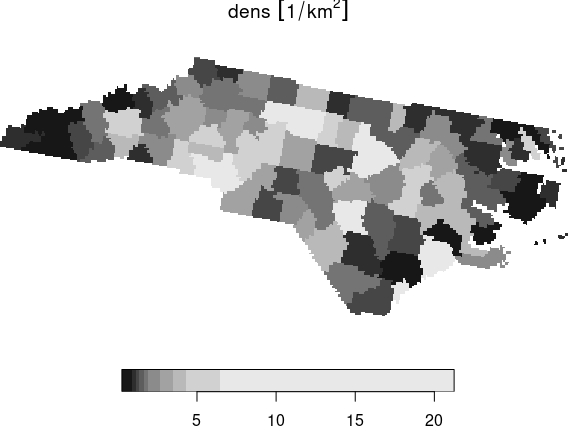This new object has a regular grid in the new CRS, aligned with the new x- and y-axes.